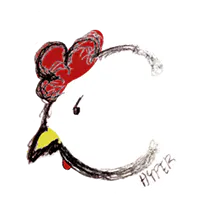# ARMin v2: Simple Robot Car and Arm Controller Using Python

Control servos/motors connected to a robot car and arm kit using a Raspberry Pi, Python, and an Xbox 360 controller.

IntermediateFull instructions provided1 hour2,291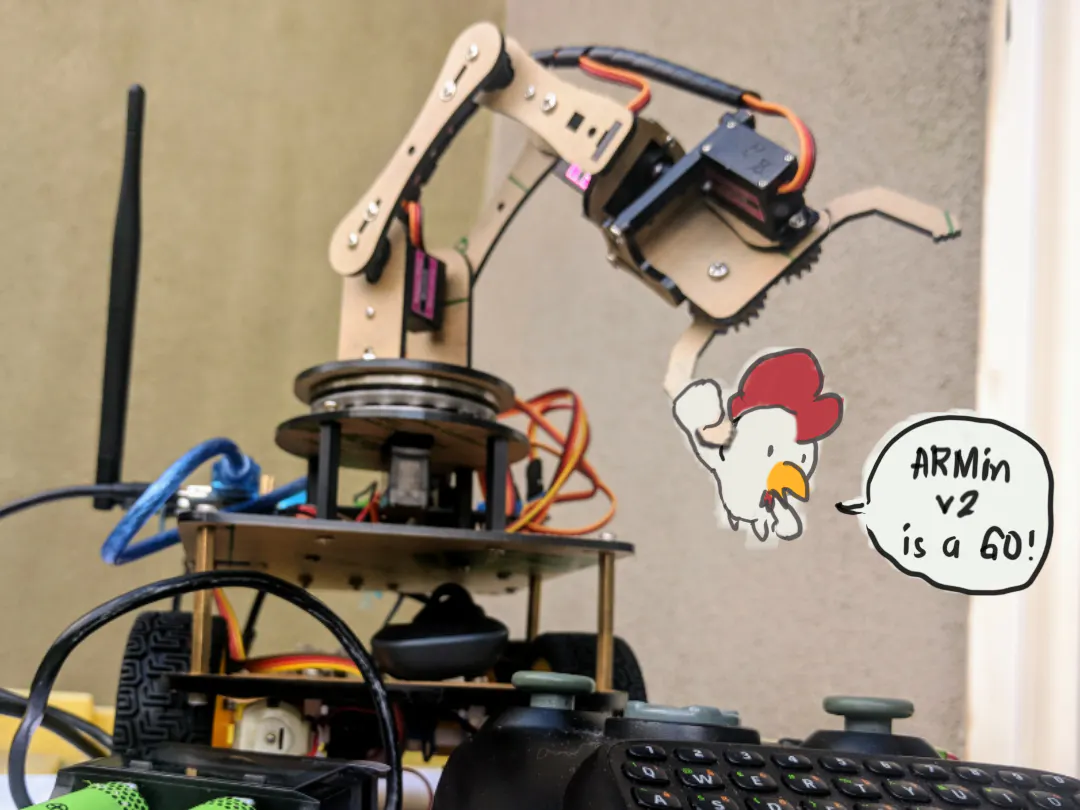## Things used in this project

### Hardware componentsArduino UNO & Genuino UNO
×1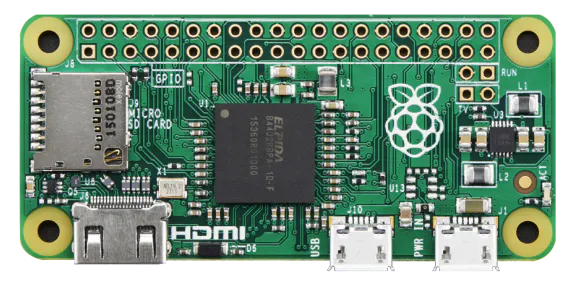Raspberry Pi Zero
×1
×1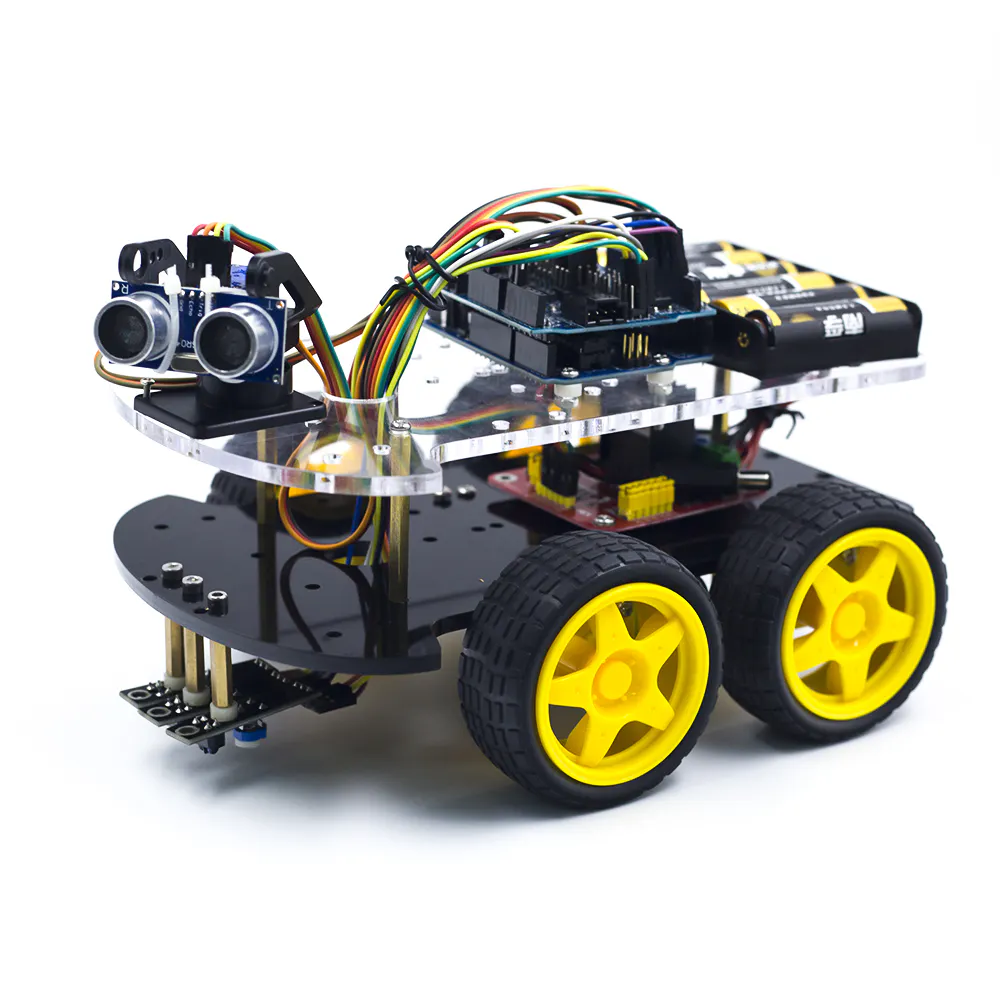Kuongshun Electronic Multi-Functional 4WD Robot Car Chassis Kit
×1
 18650 li-ion battery
×4
 xbox 360 controller
×1

### Software apps and online servicesArduino IDE

### Hand tools and fabrication machines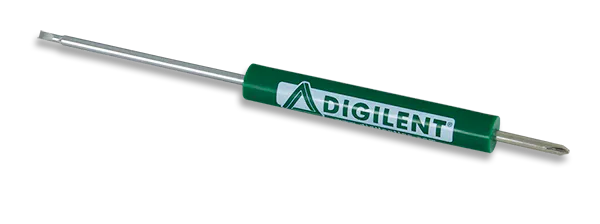Digilent Screwdriver

## Code

### arduino-control-v2.py

Python
This is the code to be run on your Raspberry Pi
```#!/usr/bin/env python
from __future__ import print_function
import xbox
from Arduino import Arduino
import time

# Define Arduino variables
servo1 = 9
servo2 = 6
servo3 = 5
servo4 = 3
servo5 = 11

IN1 = 2
IN2 = 4
IN3 = 7
IN4 = 8
ENA = 10
ENB = 12

board = Arduino()
board.Servos.attach(servo1)
board.Servos.attach(servo2)
board.Servos.attach(servo3)
board.Servos.attach(servo4)
board.Servos.attach(servo5)

board.pinMode(IN1, "OUTPUT");
board.pinMode(IN2, "OUTPUT");
board.pinMode(IN3, "OUTPUT");
board.pinMode(IN4, "OUTPUT");
board.pinMode(ENA, "OUTPUT");
board.pinMode(ENB, "OUTPUT");
board.pinMode(13, "OUTPUT")

angle1 = 90
angle2 = 90
angle3 = 90
angle4 = 90
angle5 = 0

# Format floating point number to string format -x.xxx
def fmtFloat(n):
return '{:6.3f}'.format(n)

# Print one or more values without a line feed
def show(*args):
for arg in args:
print(arg, end="")

# Print true or false value based on a boolean, without linefeed
def showIf(boolean, ifTrue, ifFalse=" "):
if boolean:
show(ifTrue)
else:
show(ifFalse)

# Move servo with respect to the left analog stick X values limited to the min-max angle set
def leftXmove(servonum,minAngle,maxAngle):
angle = angle1
if int(joy.leftX()) > 0:
angle = angle - 2
if angle <= minAngle:
angle = minAngle
return angle
elif int(joy.leftX()) < 0:
angle = angle + 2
if angle >= maxAngle:
angle = maxAngle
return angle
else:
return angle

# Move servo with respect to the left analog stick X values limited to the min-max angle set
def leftYmove(servonum,minAngle,maxAngle):
angle = angle2
if int(joy.leftY()) > 0:
angle = angle - 2
if angle <= minAngle:
angle = minAngle
return angle
elif int(joy.leftY()) < 0:
angle = angle + 2
if angle >- maxAngle:
angle = maxAngle
return angle
else:
return angle

# Move servo with respect to the right analog stick Y values limited to the min-max angle set
def rightYmove(servonum,minAngle,maxAngle):
angle = angle3
if int(joy.rightY()) > 0:
angle = angle - 2
if angle <= minAngle:
angle = minAngle
return angle
elif int(joy.rightY()) < 0:
angle = angle + 2
if angle >= maxAngle:
angle = maxAngle
return angle
else:
return angle

# Move servo with respect to the right analog stick X values limited to the min-max angle set
def rightXmove(servonum,minAngle,maxAngle):
angle = angle4
if int(joy.rightX()) > 0:
angle = angle - 2
if angle <= minAngle:
angle = minAngle
return angle
elif int(joy.rightX()) < 0:
angle = angle + 2
if angle >= maxAngle:
angle = maxAngle
return angle
else:
return angle

# Move servo when A button is pressed
def buttonA(servonum,minAngle,maxAngle):
angle5 = minAngle
if int(joy.A()) > 0:
angle5 = maxAngle
board.Servos.write(servonum,angle5)
else:
board.Servos.write(servonum,minAngle)

# Move motors when right trigger is used
def rightTrigmove():
if int(joy.rightTrigger()) > 0:
board.digitalWrite(IN1,"LOW")
board.digitalWrite(IN2,"HIGH")
board.digitalWrite(IN3,"LOW")
board.digitalWrite(IN4,"HIGH")

# Move motors when left trigger is used
def leftTrigmove():
if int(joy.leftTrigger()) > 0:
board.digitalWrite(IN1,"HIGH")
board.digitalWrite(IN2,"LOW")
board.digitalWrite(IN3,"HIGH")
board.digitalWrite(IN4,"LOW")

# Move motors when left bumper is used
def leftBumper():
if int(joy.leftBumper()) > 0:
board.digitalWrite(IN1,"HIGH")
board.digitalWrite(IN2,"LOW")
board.digitalWrite(IN3,"LOW")
board.digitalWrite(IN4,"HIGH")

# Move motors when right bumper is used
def rightBumper():
if int(joy.rightBumper()) > 0:
board.digitalWrite(IN1,"LOW")
board.digitalWrite(IN2,"HIGH")
board.digitalWrite(IN3,"HIGH")
board.digitalWrite(IN4,"LOW")

# Instantiate the controller
joy = xbox.Joystick()

# Show various axis and button states until Back button is pressed
print("Xbox controller sample: Press Back button to exit")
while not joy.Back():
# Poll controller leftX values and move servo1 accordingly
angle1 = leftXmove(servo1,0,180)
board.Servos.write(servo1,angle1)
# Poll controller leftY values and move servo2 accordingly
angle2 = leftYmove(servo2,0,120)
board.Servos.write(servo2,angle2)
# Poll controller rightY values and move servo3 accordingly
angle3 = rightYmove(servo3,0,180)
board.Servos.write(servo3,angle3)
# Poll controller rightX values and move servo4 accordingly
angle4 =  rightXmove(servo4,0,180)
board.Servos.write(servo4,angle4)
# Move claw conencted to servo5 when A is pressed
buttonA(servo5,0,90)
# Poll right trigger and move motors forward
rightTrigmove()
# Poll left trigger and move motors backward
leftTrigmove()
# Poll right bumper and turn right
rightBumper()
# Poll left bumper and turn left
leftBumper()

if (int(joy.rightTrigger()) | int(joy.leftTrigger()) | int(joy.rightBumper()) | int(joy.leftBumper()) == 0):
board.digitalWrite(IN1,"LOW")
board.digitalWrite(IN2,"LOW")
board.digitalWrite(IN3,"LOW")
board.digitalWrite(IN4,"LOW")

# Close out when done
joy.close()
```

### prototype.ino

Arduino
```#include <SoftwareSerial.h>
#include <Wire.h>
#include <Servo.h>
#include <EEPROM.h>

void Version(){
Serial.println(F("V0.6"));
}

SoftwareSerial *sserial = NULL;
Servo servos;
int servo_pins[] = {0, 0, 0, 0, 0, 0, 0, 0};
boolean connected = false;

int Str2int (String Str_value)
{
char buffer; //max length is three units
Str_value.toCharArray(buffer, 10);
int int_value = atoi(buffer);
return int_value;
}

void split(String results[], int len, String input, char spChar) {
String temp = input;
for (int i=0; i<len; i++) {
int idx = temp.indexOf(spChar);
results[i] = temp.substring(0,idx);
temp = temp.substring(idx+1);
}
}

int pinToMeasure = Str2int(data);
//  Input: Arduino pin number
//  Output: A number, from 0 to 17 expressing
//  how much capacitance is on the pin
//  When you touch the pin, or whatever you have
//  attached to it, the number will get higher
//  http://playground.arduino.cc/Code/CapacitiveSensor
//
// Variables used to translate from Arduino to AVR pin naming
volatile uint8_t* port;
volatile uint8_t* ddr;
volatile uint8_t* pin;
// Here we translate the input pin number from
//  Arduino pin number to the AVR PORT, PIN, DDR,
//  and which bit of those registers we care about.
port = portOutputRegister(digitalPinToPort(pinToMeasure));
ddr = portModeRegister(digitalPinToPort(pinToMeasure));
pin = portInputRegister(digitalPinToPort(pinToMeasure));
// Discharge the pin first by setting it low and output
delay(1);
// Make the pin an input with the internal pull-up on

// Now see how long the pin to get pulled up. This manual unrolling of the loop
// decreases the number of hardware cycles between each read of the pin,
// thus increasing sensitivity.
uint8_t cycles = 17;
if (*pin & bitmask) { cycles =  0;}
else if (*pin & bitmask) { cycles =  1;}
else if (*pin & bitmask) { cycles =  2;}
else if (*pin & bitmask) { cycles =  3;}
else if (*pin & bitmask) { cycles =  4;}
else if (*pin & bitmask) { cycles =  5;}
else if (*pin & bitmask) { cycles =  6;}
else if (*pin & bitmask) { cycles =  7;}
else if (*pin & bitmask) { cycles =  8;}
else if (*pin & bitmask) { cycles =  9;}
else if (*pin & bitmask) { cycles = 10;}
else if (*pin & bitmask) { cycles = 11;}
else if (*pin & bitmask) { cycles = 12;}
else if (*pin & bitmask) { cycles = 13;}
else if (*pin & bitmask) { cycles = 14;}
else if (*pin & bitmask) { cycles = 15;}
else if (*pin & bitmask) { cycles = 16;}

// Discharge the pin again by setting it low and output
//  It's important to leave the pins low if you want to
//  be able to touch more than 1 sensor at a time - if
//  the sensor is left pulled high, when you touch
//  two sensors, your body will transfer the charge between
//  sensors.

//return cycles;
Serial.println(cycles);
}

void Tone(String data){
int idx = data.indexOf('%');
int len = Str2int(data.substring(0,idx));
String data2 = data.substring(idx+1);
int idx2 = data2.indexOf('%');
int pin = Str2int(data2.substring(0,idx2));
String data3 = data2.substring(idx2+1);
String melody[len*2];
split(melody,len*2,data3,'%');

for (int thisNote = 0; thisNote < len; thisNote++) {
int noteDuration = 1000/Str2int(melody[thisNote+len]);
int note = Str2int(melody[thisNote]);
tone(pin, note, noteDuration);
int pause = noteDuration * 1.30;
delay(pause);
noTone(pin);
}
}

void ToneNo(String data){
int pin = Str2int(data);
noTone(pin);
}

void DigitalHandler(int mode, String data){
int pin = Str2int(data);
}else{
if(pin <0){
digitalWrite(-pin,LOW);
}else{
digitalWrite(pin,HIGH);
}
//Serial.println('0');
}
}

void AnalogHandler(int mode, String data){
int pin = Str2int(data);
}else{
String sdata;
split(sdata,2,data,'%');
int pin = Str2int(sdata);
int pv = Str2int(sdata);
analogWrite(pin,pv);
}
}

void ConfigurePinHandler(String data){
int pin = Str2int(data);
if(pin <=0){
pinMode(-pin,INPUT);
}else{
pinMode(pin,OUTPUT);
}
}

void shiftOutHandler(String data) {
String sdata;
split(sdata, 4, data, '%');
int dataPin = sdata.toInt();
int clockPin = sdata.toInt();
String bitOrderName = sdata;
byte value = (byte)(sdata.toInt());
if (bitOrderName == "MSBFIRST") {
shiftOut(dataPin, clockPin, MSBFIRST, value);
} else {
shiftOut(dataPin, clockPin, LSBFIRST, value);
}
}

void shiftInHandler(String data) {
String sdata;
split(sdata, 3, data, '%');
int dataPin = sdata.toInt();
int clockPin = sdata.toInt();
String bitOrderName = sdata;
int incoming;
if (bitOrderName == "MSBFIRST") {
incoming = (int)shiftIn(dataPin, clockPin, MSBFIRST);
} else {
incoming = (int)shiftIn(dataPin, clockPin, LSBFIRST);
}
Serial.println(incoming);
}

void SS_set(String data){
delete sserial;
String sdata;
split(sdata,3,data,'%');
int rx_ = Str2int(sdata);
int tx_ = Str2int(sdata);
int baud_ = Str2int(sdata);
sserial = new SoftwareSerial(rx_, tx_);
sserial->begin(baud_);
Serial.println("ss OK");
}

void SS_write(String data) {
int len = data.length()+1;
char buffer[len];
data.toCharArray(buffer,len);
Serial.println("ss OK");
sserial->write(buffer);
}
Serial.println(c);
}

void pulseInHandler(String data){
int pin = Str2int(data);
long duration;
if(pin <=0){
pinMode(-pin, INPUT);
duration = pulseIn(-pin, LOW);
}else{
pinMode(pin, INPUT);
duration = pulseIn(pin, HIGH);
}
Serial.println(duration);
}

void pulseInSHandler(String data){
int pin = Str2int(data);
long duration;
if(pin <=0){
pinMode(-pin, OUTPUT);
digitalWrite(-pin, HIGH);
delayMicroseconds(2);
digitalWrite(-pin, LOW);
delayMicroseconds(5);
digitalWrite(-pin, HIGH);
pinMode(-pin, INPUT);
duration = pulseIn(-pin, LOW);
}else{
pinMode(pin, OUTPUT);
digitalWrite(pin, LOW);
delayMicroseconds(2);
digitalWrite(pin, HIGH);
delayMicroseconds(5);
digitalWrite(pin, LOW);
pinMode(pin, INPUT);
duration = pulseIn(pin, HIGH);
}
Serial.println(duration);
}

String sdata;
split(sdata,3,data,'%');
int pin = Str2int(sdata);
int min = Str2int(sdata);
int max = Str2int(sdata);
int pos = -1;
for (int i = 0; i<8;i++) {
if (servo_pins[i] == pin) { //reset in place
servos[pos].detach();
servos[pos].attach(pin, min, max);
servo_pins[pos] = pin;
Serial.println(pos);
return;
}
}
for (int i = 0; i<8;i++) {
if (servo_pins[i] == 0) {pos = i;break;} // find spot in servo array
}
if (pos == -1) {;} //no array position available!
else {
servos[pos].attach(pin, min, max);
servo_pins[pos] = pin;
Serial.println(pos);
}
}

void SV_remove(String data) {
int pos = Str2int(data);
servos[pos].detach();
servo_pins[pos] = 0;
}

int pos = Str2int(data);
int angle;
Serial.println(angle);
}

void SV_write(String data) {
String sdata;
split(sdata,2,data,'%');
int pos = Str2int(sdata);
int angle = Str2int(sdata);
servos[pos].write(angle);
}

void SV_write_ms(String data) {
String sdata;
split(sdata,2,data,'%');
int pos = Str2int(sdata);
int uS = Str2int(sdata);
servos[pos].writeMicroseconds(uS);
}

void sizeEEPROM() {
Serial.println(E2END + 1);
}

void EEPROMHandler(int mode, String data) {
String sdata;
split(sdata, 2, data, '%');
if (mode == 0) {
EEPROM.write(Str2int(sdata), Str2int(sdata));
} else {
}
}

void SerialParser(void) {
// separate command from associated data

// determine command sent
if (cmd == "dw") {
DigitalHandler(1, data);
}
else if (cmd == "dr") {
DigitalHandler(0, data);
}
else if (cmd == "aw") {
AnalogHandler(1, data);
}
else if (cmd == "ar") {
AnalogHandler(0, data);
}
else if (cmd == "pm") {
ConfigurePinHandler(data);
}
else if (cmd == "ps") {
pulseInSHandler(data);
}
else if (cmd == "pi") {
pulseInHandler(data);
}
else if (cmd == "ss") {
SS_set(data);
}
else if (cmd == "sw") {
SS_write(data);
}
else if (cmd == "sr") {
}
else if (cmd == "sva") {
}
else if (cmd == "svr") {
}
else if (cmd == "svw") {
SV_write(data);
}
else if (cmd == "svwm") {
SV_write_ms(data);
}
else if (cmd == "svd") {
SV_remove(data);
}
else if (cmd == "version") {
Version();
}
else if (cmd == "to") {
Tone(data);
}
else if (cmd == "nto") {
ToneNo(data);
}
else if (cmd == "cap") {
}
else if (cmd == "so") {
shiftOutHandler(data);
}
else if (cmd == "si") {
shiftInHandler(data);
}
else if (cmd == "eewr") {
EEPROMHandler(0, data);
}
else if (cmd == "eer") {
EEPROMHandler(1, data);
}
else if (cmd == "sz") {
sizeEEPROM();
}
}

void setup()  {
Serial.begin(115200);
while (!Serial) {
; // wait for serial port to connect. Needed for Leonardo only
}
Serial.println("connected");
}

void loop() {
SerialParser();
}
```

## Credits

### HyperChiicken

10 projects • 13 followers
I cobble things together :]